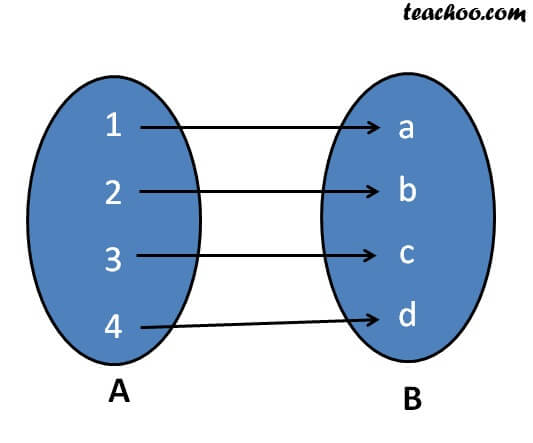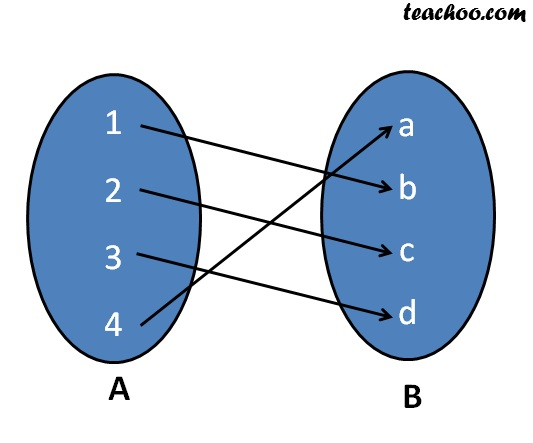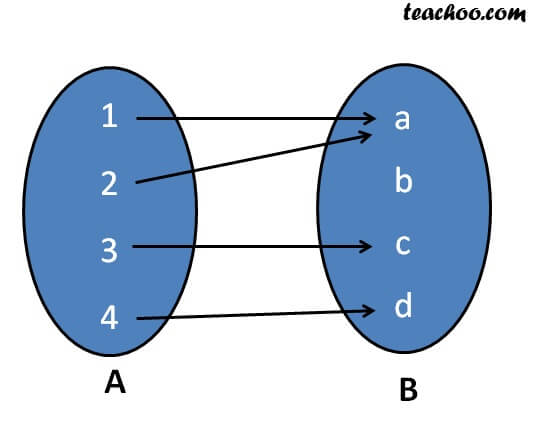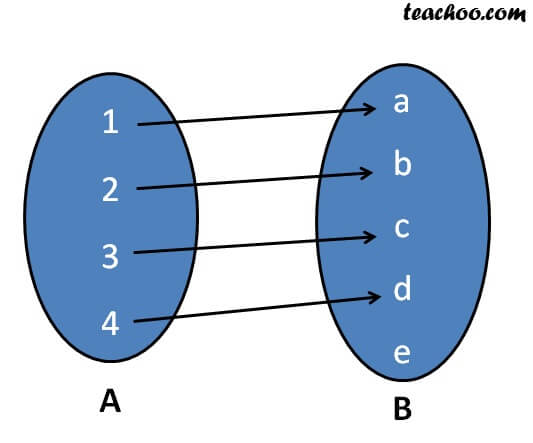To prove one-one & onto (injective, surjective, bijective)

Chapter 1 Class 12 Relation and Functions
Concept wise

f: X → Y

Function f is onto if every element of set Y has a pre-image in set X

i.e.

For every y ∈ Y,

there is x ∈ X

such that f(x) = y

### How to check if function is onto - Method 1

In this method, we check for each and every element manually if it has unique image

Check whether the following are onto?Since all elements of set B has a pre-image in set A,

it is ontoSince all elements of set B has a pre-image in set A,

it is ontoSince element b has no pre-image,

it is not ontoSince element e has no pre-image,

it is not onto

### How to check if function is onto -  Method 2

This method is used if there are large numbers

Example:

f : N N   (There are infinite number of natural numbers)

f : R R   (There are infinite number of real numbers )

f : Z Z    (There are infinite number of integers)

Steps :

How to check onto?

1. Put y =  f(x)
2. Find x in terms of y.

If x ∈ X, then f is onto

Let’s take some examples

f: R R

f(x) = x

Is f onto?

-a-

1. Put y = f(x)
2. Find x in terms of y.

If x ∈ X, then f is onto

y = f(x)

y = x

∴ x = y

Since y ∈ R

x  = y also belongs to R

i.e. x ∈ R

∴ f is onto

-ea-

f: R → R

f(x) = 1

Is f onto?

-a-

f(x) = 1

∴ y = 1

So, value of y will always be 1

∴ There is no value x where y = 2

⇒ 2 does not have a pre-image in X

∴ f is not onto

-ea-

Learn in your speed, with individual attention - Teachoo Maths 1-on-1 Class